# Samacheer Kalvi 9th Maths Solutions Chapter 2 Real Numbers Ex 2.6

## Tamilnadu Samacheer Kalvi 9th Maths Solutions Chapter 2 Real Numbers Ex 2.6

Ex 2.6 Class 9 Question 1.
Simplify the following using addition and subtraction properties of surds:
(i) 5$$\sqrt { 3 }$$ + 18$$\sqrt { 3 }$$ – 2$$\sqrt{3}$$
(ii) 4$$\sqrt [ 3 ]{ 5 }$$ + 2$$\sqrt [ 2 ]{ 5 }$$ – 3$$\sqrt [ 3 ]{ 5 }$$
(iii) 3$$\sqrt { 75 }$$ + 5$$\sqrt { 48 }$$ – $$\sqrt { 243 }$$
(iv) 5$$\sqrt [ 3 ]{ 40 }$$ + 2$$\sqrt [ 3 ]{ 625 }$$ – 3$$\sqrt [ 3 ]{ 320 }$$
Solution:
(i) 5$$\sqrt { 3 }$$ + 18$$\sqrt { 3 }$$ – 2$$\sqrt { 3 }$$ = (5 + 18 – 2)$$\sqrt { 3 }$$ = 21$$\sqrt { 3 }$$
(ii) 4$$\sqrt [ 3 ]{ 5 }$$ + 2$$\sqrt [ 2 ]{ 5 }$$ – 3$$\sqrt [ 3 ]{ 5 }$$ = (4 + 2 – 3)$$\sqrt [ 3 ]{ 5 }$$ = 3$$\sqrt [ 3 ]{ 5 }$$
(iii) 3$$\sqrt { 75 }$$ + 5$$\sqrt { 48 }$$ – $$\sqrt { 243 }$$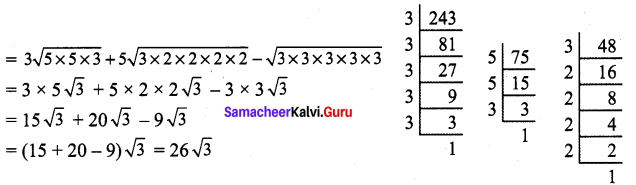(iv) 5$$\sqrt [ 3 ]{ 40 }$$ + 2$$\sqrt [ 3 ]{ 625 }$$ – 3$$\sqrt [ 3 ]{ 320 }$$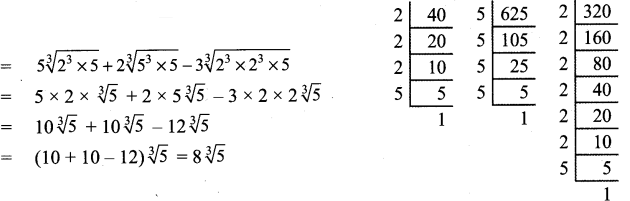9th Class Maths Exercise 2.6 Solution Question 2.
Simplify the following using multiplication and division properties of surds :
(i) $$\sqrt{3} \times \sqrt{5} \times \sqrt{2}$$
(ii) $$\sqrt { 35 }$$ ÷ $$\sqrt { 7 }$$
(iii) $$\sqrt{27} \times \sqrt{8} \times \sqrt{125}$$
(iv) (7$$\sqrt { a }$$ – 5$$\sqrt { b }$$)(7$$\sqrt { a }$$ + 5$$\sqrt { b }$$)
(v) $$[\sqrt{\frac{225}{729}}-\sqrt{\frac{25}{144}}] \div \sqrt{\frac{16}{81}}$$
Solution: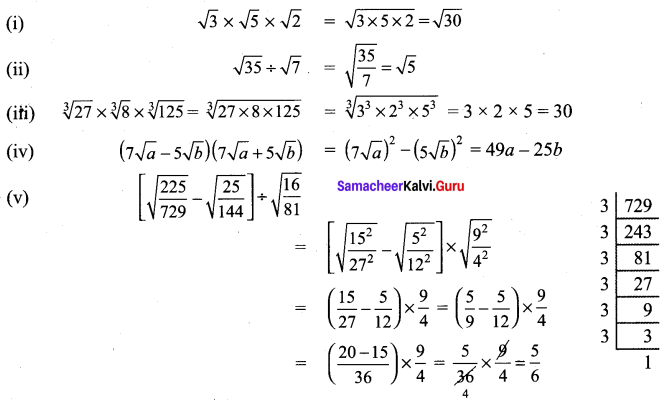9th Maths Exercise 2.6 Question 3.
If $$\sqrt { 7 }$$ =1.414, $$\sqrt { 3 }$$ = 1.732, $$\sqrt { 5 }$$ = 2.236, $$\sqrt { 10 }$$ = 3.162, then find the values of the following correct to 3 places of decimals.
(i) $$\sqrt{40}-\sqrt{20}$$
(ii) $$\sqrt{300}+\sqrt{90}-\sqrt{8}$$
Solution: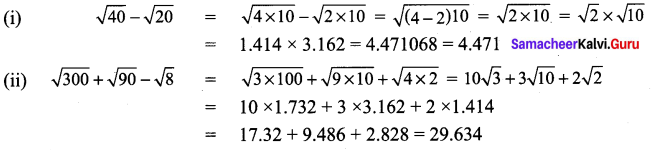2.6 9th Class Math Question 4.
Arrange surds in descending order :
(i) $$\sqrt{5}, \sqrt{4}, \sqrt{3}$$
(ii) $$\sqrt{\sqrt{5}}, \sqrt{\sqrt{7}}, \sqrt{\sqrt{3}}$$
Solution:
(i) $$\sqrt{5}, \sqrt{4}, \sqrt{3}$$
5$$\frac { 1 }{ 3 }$$
∴ The order of the surds $$\sqrt{5}, \sqrt{4}, \sqrt{3}$$ are 3, 9, 6.
4$$\frac { 1 }{ 9 }$$
3$$\frac { 1 }{ 6 }$$ l.c.m of 3, 9, 6 is 18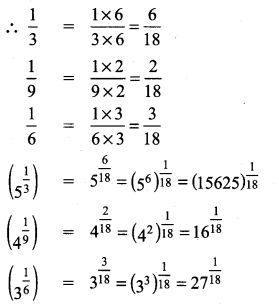∴ The descending order of $$\sqrt{5}, \sqrt{4}, \sqrt{3}$$ is $$(15625)^{\frac{1}{18}}>(27)^ {\frac{1}{18}} >16^{\frac{1}{18}} \text { i.e. } \sqrt{5}>\sqrt{3}>\sqrt{4}$$

(ii) $$\sqrt{\sqrt{5}}, \sqrt{\sqrt{7}}, \sqrt{\sqrt{3}}$$
The order of the surds $$\sqrt{\sqrt{5}}, \sqrt{\sqrt{7}}, \sqrt{\sqrt{3}}$$ are 6, 12, 4
l.c.m of 6, 12, 4 is 12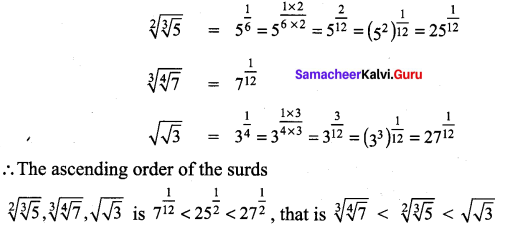Maths Class 9 Exercise 2.6 Solutions Question 5.
Can you get a pure surd when you find
(i) the sum of two surds
(ii) the difference of two surds
(iii) the product of two surds
(iv) the quotient of two surds
Justify each answer with an example.
Solution: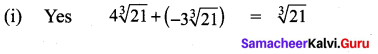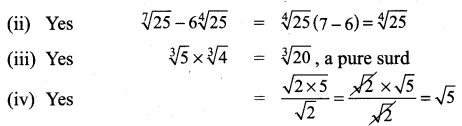Class 9 Maths Ex 2.6 Solution Question 6.
Can you get a rational number when you compute
(i) the sum of two surds
(ii) the difference of two surds
(iii) the product of two surds
(iv) the quotient of two surds
Justify each answer with an example.
Solution:
(i) Yes (5 – $$\sqrt{3}$$) + (5 + $$\sqrt{3}$$) = 10, a rational number
(ii) Yes $$(5+\sqrt{7})-(-6+\sqrt{7})=11$$, a rational number
(iii) Yes (5 + $$\sqrt{3}$$ ) (5 – $$\sqrt{3}$$ ) = 25 – 3 = 22, a rational number
(iv) Yes $$\frac{5 \sqrt{3}}{\sqrt{3}}=5$$ ,a rational number